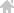# 和平精英SS16赛季上分攻略 吃鸡SS16赛季手册冲分技巧分享[多图](20*4+40*2+80*2)+80=400积分。▲参赛挑战▲活跃挑战

(25+50+75+100+125)*16+200=6200

(40+60+80+100+120)*4+200=1800

6200+1800=8000积分。